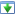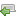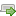Choose a version here. If you've not already started the tutorial, just go with the latest one.

 30 Aug 2014 Preview version Choose 5 Oct 2014 Explicitly adds a specific charset for htmlspecialchars(), and wraps it with a custom function Choose 16 Oct 2014 Updated redirect function to work with vhost subfolders Choose 4 Nov 2014 Minor improvements: added missing docblock, fixed security issue, CSS tweak. Switched hashing method to DEFAULT instead of BCRYPT, this is best practice. Choose 25 Nov 2014 Improve the notes on getting started, in particular choosing a programmer's editor. Added introduction to mod_rewrite rules. Choose 16 Aug 2018 Some bug fixes, remove compatibility library for earlier version of PHP Choose ♥
OK
NB: There are several versions of this tutorial, each successive one containing additional improvements. If you're in the middle of working through it, please check the versions panel above, to ensure you're not mixing code from different versions.

The next thing I decided to implement was a log-on system. This will allow a blog administrator to write, edit and delete posts, and to delete comments. In this set of changes, I add a users table, and allow the installer to create an admin user with a new password each time it is run. So, make the following changes, and then re-create the database:`93`
`94`
`95`
`96`
` 'http://anotherexample.com/',`
` "This is a comment from Jonny"`
` )`
`;`
`93`
`94`
`95`
`96`
`97`
`98`
`99`
`100`
`101`
`102`
`103`
`104`
` 'http://anotherexample.com/',`
` "This is a comment from Jonny"`
` )`
`;`
`CREATE TABLE user (`
` id INTEGER PRIMARY KEY AUTOINCREMENT NOT NULL,`
` username VARCHAR NOT NULL,`
` password VARCHAR NOT NULL,`
` created_at VARCHAR NOT NULL,`
` is_enabled BOOLEAN NOT NULL DEFAULT true`
`);`
`10`
`11`
`12`
`13`
` `
` `
` `
` `
` `
` `
` `
` `
` `
` `
` `
` `
` `
`14`
`15`
`16`
`31`
`32`
`33`
`34`
`35`
`36`
`37`
`38`
` `
` `
`39`
`40`
`41`
`42`
` `
` `
` `
`43`
`44`
`45`
`78`
`79`
`80`
` `
`81`
`82`
`83`
`88`
`89`
`90`
` `
` `
` `
` `
` `
`91`
`92`
`93`
`{`
` // Here's the install`
` \$pdo = getPDO();`
` list(\$_SESSION['count'], \$_SESSION['error']) = installBlog(\$pdo);`
` // ... and here we redirect from POST to GET`
` redirectAndExit('install.php');`
`// Let's see if we've just installed`
`\$attempted = false;`
`if (\$_SESSION)`
`{`
` \$attempted = true;`
` \$count = \$_SESSION['count'];`
` \$error = \$_SESSION['error'];`
` // Unset session variables, so we only report the install/failure once`
` unset(\$_SESSION['count']);`
` unset(\$_SESSION['error']);`
`}`
`?>`
` <div class="success box">`
` The database and demo data was created OK.`
` <?php foreach (array('post', 'comment') as \$tableName): ?>`
` <?php if (isset(\$count[\$tableName])): ?>`
` <?php // Prints the count ?>`
` were created.`
` <?php endif ?>`
` <?php endforeach ?>`
` </div>`
` <p>`
`10`
`11`
`12`
`13`
`14`
`15`
`16`
`17`
`18`
`19`
`20`
`21`
`22`
`23`
`24`
`25`
`26`
`27`
`28`
`29`
`31`
`32`
`33`
`34`
`35`
`36`
`37`
`38`
`39`
`40`
`41`
`42`
`43`
`44`
`45`
`46`
`47`
`48`
`49`
`50`
`78`
`79`
`80`
`81`
`82`
`83`
`84`
`88`
`89`
`90`
`91`
`92`
`93`
`94`
`95`
`96`
`97`
`98`
`{`
` // Here's the install`
` \$pdo = getPDO();`
` list(\$rowCounts, \$error) = installBlog(\$pdo);`
` \$password = '';`
` if (!\$error)`
` {`
` \$username = 'admin';`
` list(\$password, \$error) = createUser(\$pdo, \$username);`
` }`
` \$_SESSION['count'] = \$rowCounts;`
` \$_SESSION['error'] = \$error;`
` \$_SESSION['username'] = \$username;`
` \$_SESSION['password'] = \$password;`
` \$_SESSION['try-install'] = true;`
` // ... and here we redirect from POST to GET`
` redirectAndExit('install.php');`
`// Let's see if we've just installed`
`\$attempted = false;`
`if (isset(\$_SESSION['try-install']))`
`{`
` \$attempted = true;`
` \$count = \$_SESSION['count'];`
` \$error = \$_SESSION['error'];`
` \$username = \$_SESSION['username'];`
` \$password = \$_SESSION['password'];`
` // Unset session variables, so we only report the install/failure once`
` unset(\$_SESSION['count']);`
` unset(\$_SESSION['error']);`
` unset(\$_SESSION['username']);`
` unset(\$_SESSION['password']);`
` unset(\$_SESSION['try-install']);`
`}`
`?>`
` <div class="success box">`
` The database and demo data was created OK.`
` <?php // Report the counts for each table ?>`
` <?php foreach (array('post', 'comment') as \$tableName): ?>`
` <?php if (isset(\$count[\$tableName])): ?>`
` <?php // Prints the count ?>`
` were created.`
` <?php endif ?>`
` <?php endforeach ?>`
` <?php // Report the new password ?>`
` The new '<?php echo htmlEscape(\$username) ?>' password is`
` <span style="font-size: 1.2em;"><?php echo htmlEscape(\$password) ?></span>`
` (copy it to clipboard if you wish).`
` </div>`
` <p>`
`72`
`73`
`74`
` return array(\$count, \$error);`
`}`
`72`
`73`
`74`
`75`
`76`
`77`
`78`
`79`
`80`
`81`
`82`
`83`
`84`
`85`
`86`
`87`
`88`
`89`
`90`
`91`
`92`
`93`
`94`
`95`
`96`
`97`
`98`
`99`
`100`
`101`
`102`
`103`
`104`
`105`
`106`
`107`
`108`
`109`
`110`
`111`
`112`
`113`
`114`
`115`
`116`
`117`
`118`
`119`
`120`
`121`
`122`
`123`
`124`
`125`
`126`
`127`
`128`
`129`
`130`
`131`
`132`
`133`
`134`
`135`
`136`
` return array(\$count, \$error);`
`}`
`/**`
` * Creates a new user in the database`
` *`
` * @param PDO \$pdo`
` * @param string \$username`
` * @param integer \$length`
` * @return array Duple of (password, error)`
` */`
`function createUser(PDO \$pdo, \$username, \$length = 10)`
`{`
` // This algorithm creates a random password`
` \$alphabet = range(ord('A'), ord('z'));`
` \$alphabetLength = count(\$alphabet);`
` \$password = '';`
` for(\$i = 0; \$i < \$length; \$i++)`
` {`
` \$letterCode = \$alphabet[rand(0, \$alphabetLength - 1)];`
` \$password .= chr(\$letterCode);`
` }`
` \$error = '';`
` // Insert the credentials into the database`
` \$sql = "`
` INSERT INTO`
` user`
` (username, password, created_at)`
` VALUES (`
` :username, :password, :created_at`
` )`
` ";`
` \$stmt = \$pdo->prepare(\$sql);`
` if (\$stmt === false)`
` {`
` \$error = 'Could not prepare the user creation';`
` }`
` // We're storing the password in plaintext, will fix that later`
` if (!\$error)`
` {`
` \$result = \$stmt->execute(`
` array(`
` 'username' => \$username,`
` 'password' => \$password,`
` 'created_at' => getSqlDateForNow(),`
` )`
` );`
` if (\$result === false)`
` {`
` \$error = 'Could not run the user creation';`
` }`
` }`
` if (\$error)`
` {`
` \$password = '';`
` }`
` return array(\$password, \$error);`
`}`

There are two fields in the new user table that I added based on my experience rather than an immediate need. These are `created_at`, which holds the date and time when the user was first set up, and `is_enabled`, which allows us to turn users on and off. Most user systems will find a practical use for these simple features during their lifetime.

You'll have noticed a comment in lib/install.php noting that the password is stored in plaintext. This means that, as it stands, passwords would be stored literally, which is considered to be very bad practice indeed. What we should do is to store passwords in an encoded, non-reversible format, so that even if they are stolen they will be nearly impossible to read. This acts as a form of protection should a cracker get through our security and steal our database.

So, let's make that improvement straight away. Here are the changes:`111`
`112`
`113`
`114`
` `
` `
` `
` `
` `
` `
` `
` `
` `
` `
`115`
`116`
`117`
`118`
`119`
`120`
`121`
`122`
`123`
` \$error = 'Could not prepare the user creation';`
` }`
` // We're storing the password in plaintext, will fix that later`
` if (!\$error)`
` {`
` \$result = \$stmt->execute(`
` array(`
` 'username' => \$username,`
` 'password' => \$password,`
` 'created_at' => getSqlDateForNow(),`
` )`
` );`
`111`
`112`
`113`
`114`
`115`
`116`
`117`
`118`
`119`
`120`
`121`
`122`
`123`
`124`
`125`
`126`
`127`
`128`
`129`
`130`
`131`
`132`
`133`
` \$error = 'Could not prepare the user creation';`
` }`
` if (!\$error)`
` {`
` // Create a hash of the password, to make a stolen user database (nearly) worthless`
` \$hash = password_hash(\$password, PASSWORD_DEFAULT);`
` if (\$hash === false)`
` {`
` \$error = 'Password hashing failed';`
` }`
` }`
` // Insert user details, including hashed password`
` if (!\$error)`
` {`
` \$result = \$stmt->execute(`
` array(`
` 'username' => \$username,`
` 'password' => \$hash,`
` 'created_at' => getSqlDateForNow(),`
` )`
` );`

So, what does the new code do? It makes use of a PHP function called `password_hash()`, which takes a password as input and produces what is known as a hash. A hash is a mathematical calculation that is strictly one way, which means that if sometimes steals our database of password hashes, they will find it very difficult indeed to recreate the passwords they were generated from.

The next step is to add a login form, and a link from which to access it:`1`
`2`
`3`
`4`
`5`
`6`
`7`
`8`
`9`
`10`
`11`
`12`
`13`
`14`
`15`
`16`
`17`
`18`
`19`
`20`
`21`
`22`
`23`
`24`
`25`
`26`
`27`
`28`
`<!DOCTYPE html>`
`<html>`
` <head>`
` <title>`
` A blog application | Login`
` </title>`
` <meta http-equiv="Content-Type" content="text/html;charset=utf-8" />`
` </head>`
` <body>`
` <?php require 'templates/title.php' ?>`
` <p>Login here:</p>`
` <form`
` method="post"`
` >`
` <p>`
` Username:`
` <input type="text" name="username" />`
` </p>`
` <p>`
` Password:`
` <input type="password" name="password" />`
` </p>`
` <input type="submit" name="submit" value="Login" />`
` </form>`
` </body>`
`</html>`
` `
` `
` `
` `
`1`
`2`
`3`
`<a href="index.php">`
` <h1>Blog title</h1>`
`</a>`
`1`
`2`
`3`
`4`
`5`
`6`
`7`
`<div style="float: right;">`
` <a href="login.php">Log in</a>`
`</div>`
`<a href="index.php">`
` <h1>Blog title</h1>`
`</a>`

In the next change, we add our familiar block of business logic before the main HTML. In this case, it checks to see if the form has been submitted; if it has, then it turns on the session system (more about that in a minute), creates a hash of the submitted password, and compares it with the hash stored in the database.

If the user gets their username wrong (e.g. it is not found) or the password wrong (the password hash does not match the one for the username supplied) then we regard this as a login failure. It is important for us not to be too helpful here (such as explaining that a username does not exist), as this information might be useful to a system cracker.

If the password matches however, then we call `login()` to sign in the user, and then we redirect to the home page using `redirectAndExit()`.

So, with that explained, let's add the changes:`139`
`140`
`141`
` return \$stmt->fetchAll(PDO::FETCH_ASSOC);`
`}`
`139`
`140`
`141`
`142`
`143`
`144`
`145`
`146`
`147`
`148`
`149`
`150`
`151`
`152`
`153`
`154`
`155`
`156`
`157`
`158`
`159`
`160`
`161`
`162`
`163`
`164`
`165`
`166`
`167`
`168`
`169`
`170`
`171`
`172`
`173`
`174`
`175`
`176`
`177`
`178`
`179`
` return \$stmt->fetchAll(PDO::FETCH_ASSOC);`
`}`
`function tryLogin(PDO \$pdo, \$username, \$password)`
`{`
` \$sql = "`
` SELECT`
` password`
` FROM`
` user`
` WHERE`
` username = :username`
` ";`
` \$stmt = \$pdo->prepare(\$sql);`
` \$stmt->execute(`
` array('username' => \$username, )`
` );`
` // Get the hash from this row, and use the third-party hashing library to check it`
` \$hash = \$stmt->fetchColumn();`
` \$success = password_verify(\$password, \$hash);`
` return \$success;`
`}`
`/**`
` * Logs the user in`
` *`
` * For safety, we ask PHP to regenerate the cookie, so if a user logs onto a site that a cracker`
` * has prepared for him/her (e.g. on a public computer) the cracker's copy of the cookie ID will be`
` * useless.`
` *`
` * @param string \$username`
` */`
`function login(\$username)`
`{`
` session_regenerate_id();`
` \$_SESSION['logged_in_username'] = \$username;`
`}`
` `
` `
` `
` `
` `
` `
` `
` `
` `
` `
` `
` `
` `
` `
` `
` `
` `
` `
` `
` `
` `
` `
` `
` `
` `
` `
` `
` `
` `
`1`
`2`
`3`
`38`
`39`
`40`
` `
` `
` `
` `
` `
` `
` `
`41`
`42`
`43`
`52`
`53`
`54`
`55`
` `
` `
` `
` `
`56`
`57`
`58`
`<!DOCTYPE html>`
`<html>`
` <head>`
` <body>`
` <?php require 'templates/title.php' ?>`
` <p>Login here:</p>`
` <form`
` >`
` <p>`
` Username:`
` <input type="text" name="username" />`
` </p>`
` <p>`
` Password:`
`1`
`2`
`3`
`4`
`5`
`6`
`7`
`8`
`9`
`10`
`11`
`12`
`13`
`14`
`15`
`16`
`17`
`18`
`19`
`20`
`21`
`22`
`23`
`24`
`25`
`26`
`27`
`28`
`29`
`30`
`31`
`32`
`38`
`39`
`40`
`41`
`42`
`43`
`44`
`45`
`46`
`47`
`48`
`49`
`50`
`52`
`53`
`54`
`55`
`56`
`57`
`58`
`59`
`60`
`61`
`62`
`<?php`
`require_once 'lib/common.php';`
`// We need to test for a minimum version of PHP, because earlier versions have bugs that affect security`
`if (version_compare(PHP_VERSION, '5.3.7') < 0)`
`{`
` throw new Exception(`
` 'This system needs PHP 5.3.7 or later'`
` );`
`}`
`// Handle the form posting`
`\$username = '';`
`if (\$_POST)`
`{`
` // Init the session and the database`
` session_start();`
` \$pdo = getPDO();`
` // We redirect only if the password is correct`
` \$username = \$_POST['username'];`
` \$ok = tryLogin(\$pdo, \$username, \$_POST['password']);`
` if (\$ok)`
` {`
` login(\$username);`
` redirectAndExit('index.php');`
` }`
`}`
`?>`
`<!DOCTYPE html>`
`<html>`
` <head>`
` <body>`
` <?php require 'templates/title.php' ?>`
` <?php // If we have a username, then the user got something wrong, so let's have an error ?>`
` <?php if (\$username): ?>`
` <div style="border: 1px solid #ff6666; padding: 6px;">`
` The username or password is incorrect, try again`
` </div>`
` <?php endif ?>`
` <p>Login here:</p>`
` <form`
` >`
` <p>`
` Username:`
` <input`
` type="text"`
` name="username"`
` value="<?php echo htmlEscape(\$username) ?>"`
` />`
` </p>`
` <p>`
` Password:`

Now we have a way to determine whether users are logged in or not, let's switch that feature on for all pages. Here we also modify the HTML snippet that contains the page header, so it can show the appropriate login/logout link.`1`
`2`
`3`
` `
` `
`4`
`5`
`6`
`<?php`
`require_once 'lib/common.php';`
`// Connect to the database, run a query, handle errors`
`\$pdo = getPDO();`
`\$stmt = \$pdo->query(`
`1`
`2`
`3`
`4`
`5`
`6`
`7`
`8`
`<?php`
`require_once 'lib/common.php';`
`session_start();`
`// Connect to the database, run a query, handle errors`
`\$pdo = getPDO();`
`\$stmt = \$pdo->query(`
`177`
`178`
`179`
` \$_SESSION['logged_in_username'] = \$username;`
`}`
`177`
`178`
`179`
`180`
`181`
`182`
`183`
`184`
` \$_SESSION['logged_in_username'] = \$username;`
`}`
`function isLoggedIn()`
`{`
` return isset(\$_SESSION['logged_in_username']);`
`}`
`9`
`10`
`11`
` `
` `
`12`
`13`
`14`
`15`
`16`
`17`
`18`
`19`
`20`
` );`
`}`
`// Handle the form posting`
`\$username = '';`
`if (\$_POST)`
`{`
` // Init the session and the database`
` session_start();`
` \$pdo = getPDO();`
` // We redirect only if the password is correct`
`9`
`10`
`11`
`12`
`13`
`14`
`15`
`16`
`17`
`18`
` `
`19`
`20`
`21`
` );`
`}`
`session_start();`
`// Handle the form posting`
`\$username = '';`
`if (\$_POST)`
`{`
` // Init the database`
` \$pdo = getPDO();`
` // We redirect only if the password is correct`
`1`
`2`
` `
` `
` `
` `
`3`
`4`
`5`
`<div style="float: right;">`
` <a href="login.php">Log in</a>`
`</div>`
`<a href="index.php">`
`1`
`2`
`3`
`4`
`5`
`6`
`7`
`8`
`9`
`<div style="float: right;">`
` <?php if (isLoggedIn()): ?>`
` <a href="logout.php">Log out</a>`
` <?php else: ?>`
` <a href="login.php">Log in</a>`
` <?php endif ?>`
`</div>`
`<a href="index.php">`
`2`
`3`
`4`
` `
` `
`5`
`6`
`7`
`require_once 'lib/common.php';`
`require_once 'lib/view-post.php';`
`// Get the post ID`
`if (isset(\$_GET['post_id']))`
`{`
`2`
`3`
`4`
`5`
`6`
`7`
`8`
`9`
`require_once 'lib/common.php';`
`require_once 'lib/view-post.php';`
`session_start();`
`// Get the post ID`
`if (isset(\$_GET['post_id']))`
`{`

You may have noticed, if you tried the logout link, that this page does not yet exist. So let's add that now in the following set of changes. Whilst we are at it, let's greet the user by their username while they are logged in, as this makes the experience of the site a bit more friendly.`178`
`179`
`180`
` `
` `
` `
` `
` `
` `
` `
` `
` `
` `
` `
` `
` `
`181`
`182`
`183`
` \$_SESSION['logged_in_username'] = \$username;`
`}`
`function isLoggedIn()`
`{`
` return isset(\$_SESSION['logged_in_username']);`
`178`
`179`
`180`
`181`
`182`
`183`
`184`
`185`
`186`
`187`
`188`
`189`
`190`
`191`
`192`
`193`
`194`
`195`
`196`
` \$_SESSION['logged_in_username'] = \$username;`
`}`
`/**`
` * Logs the user out`
` */`
`function logout()`
`{`
` unset(\$_SESSION['logged_in_username']);`
`}`
`function getAuthUser()`
`{`
` return isLoggedIn() ? \$_SESSION['logged_in_username'] : null;`
`}`
`function isLoggedIn()`
`{`
` return isset(\$_SESSION['logged_in_username']);`
`1`
`2`
`3`
`4`
`5`
`6`
`<?php`
`require_once 'lib/common.php';`
`session_start();`
`logout();`
`redirectAndExit('index.php');`
`1`
`2`
` `
`3`
`4`
`5`
`<div style="float: right;">`
` <?php if (isLoggedIn()): ?>`
` <a href="logout.php">Log out</a>`
` <?php else: ?>`
` <a href="login.php">Log in</a>`
`1`
`2`
`3`
`4`
`5`
`6`
`<div style="float: right;">`
` <?php if (isLoggedIn()): ?>`
` Hello <?php echo htmlEscape(getAuthUser()) ?>.`
` <a href="logout.php">Log out</a>`
` <?php else: ?>`
` <a href="login.php">Log in</a>`

That's all for this chapter, so give your application a good test, especially the new login and logout features. When you're ready, we'll proceed in the next chapter with some small tweaks to improve what we have.